1:10 AM
Maupertuis' Principle: Minimum Action Path at Fixed Energy

# Maupertuis' Principle: Minimum Action Path at Fixed Energy

### Divine Guidance

Incredibly, Maupertuis came up with a kind of principle of least action in 1747, long before the work of Lagrange and Hamilton. Maupertuis thought a body moved along a path such that the sum of products of mass, speed and displacement taken over time was minimized, and he saw that as the hand of God at work. This didn't go over well with his skeptical fellow countrymen, such as Voltaire, and in fact his formulation wasn't quite right, but history has given him partial credit, his name on a least action principle.

Suppose we are considering the motion of a particle moving around in a plane from some initial point and timeto some final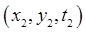. Suppose its potential energy is a function of position,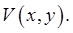For example, imagine aiming for the hole on a rather bumpy putting green, but also requiring that the ball take a definite time, say two seconds, from being hit to falling in the hole. The action principle we've talked about so far will give the path, parameterized by time,But that's not what you're interested in doing! Of course you want the ball to get in the hole, but you're not obsessed with how long it takes to get there. Yet without that time requirement, there are obviously many possible paths. If you hit it really fast, so its kinetic energy is far greater than the gravitational potential energy variations in the bumpy green, it will go close to a straight line (we're assuming that when it gets over the hole, it will drop in). As you slow down, the winning path will deviate from a straight line because of the uneven terrain. So the physical path to the hole will vary continuously with initial kinetic energy.

Maupertuis' principle is about what is the pathto the hole, say fromto, for a given initial energy.

So now we're fixing the beginning and end points in space, but allowing possible variation in the final time. Also, we're fixing the energy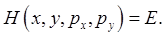This means that in varying the path to minimize the action, we must restrict ourselves to the class of paths having energyIn the bumpy putting green, you're giving the ball a fixed initial speed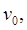and trying different initial directions to get it in the hole.

From the expression for the differential of action in terms of varying the endpoint (as well as the rest of the path -- remember, that gives the integral term that disappears along the dynamical path), we have all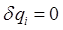(the endpoint is fixed at the hole), leaving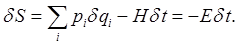(Since we're restricting to paths with energycan be replaced by.)

### The Abbreviated Action

Writing the action in the integral form along this constant energy path, we can trivially do the time integral: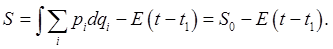Therefore, from the resultit necessarily follows that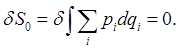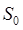is called the abbreviated action: this is Maupertuis' principle.

The abbreviated action for the physical path is the minimum among all paths satisfying energy conservation with total energyand passing through the designated final point -- we don't care when. Note that not all values ofwill work -- for example, if we start putting the ball from a low point in the green, we'll need to give it enough energy to get out of the hollow to begin with. But there will be valid physical paths for a wide range of energy values, since the final arrival time is flexible.

Naturally, since this is a path through configuration space, to evaluate the abbreviated actionit must be expressed in terms of the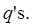For the usual Lagrangianwith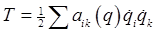, and momentawe find the abbreviated actionThis is indeed an integral along a path in configuration space, but we need to get rid of the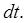Physically, we can see how to do this -- since we know the total energy, the kinetic energy at a point is, so that determines the speed, hence the time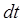to move by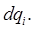That is, (following Landau),

from which.

(This doesn't look like a very healthy mathematical object, but as you'll see, it's fine.)

HenceTo take a very simple case; if there is no potential, and just a free particle,this is nothing but the length of the path multiplied by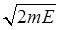, minimized by a straight line between the two points.

If we have a particle of massin a spatially varying potential, the abbreviated action reduces to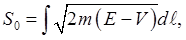where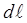is an element of path length. (This is obvious, really -- the square root is the absolute value of the momentum, and the momentum vector, of course, points along the path.)

The matrix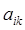, sometimes called the mass matrix, is evidently a metric, a measure in the configuration space, by which the "length" of the paths, and particularly the minimum action path, are measured.

Exercise: use Maupertuis' principle to find the path of a cannonball, energyfired at a target which is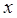meters distant horizontally, both cannon and target being at sea level (think ships!).

### Maupertuis' Principle and the Time-Independent Schrodinger Equation

Recall that the action, multiplied byis equivalent to the phase in quantum mechanics. The case we're discussing here is evidently related to the time-independent Schrodinger equation, the one for an energy eigenstate, with the time-dependent phase factored out. In other words, imagine solving the time-independent Schrodinger equation for a particle in a potential by summing over paths. In the classical limit, the abbreviated action gives the total phase change along a path. Minimizing this to find the classical path exactly parallels our earlier discussion of Fermat's principle of least time, paths close to Maupertuis' minimum have total phase change along them all the same to leading order, and so add coherently.

Category: Education | Views: 298 | Added by: farrel | | Rating: 0.0/0